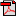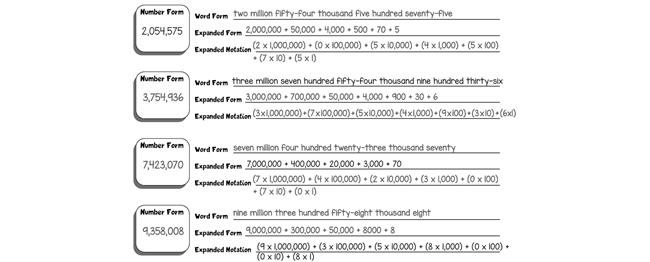# Lesson 1: Numbers to 1,000,000 Review

## Activities

### Activity 1: Working With Big Numbers

You will test your knowledge of place value by completing the "Big Numbers" sheet. When you are finished, read the numbers aloud to a parent.Student Activity Page
During this activity, your child will answer questions about two big numbers. Be ready to check his understanding of place value and adding multiples of 10 in big numbers once he completes the assigned sheet.
Allow your child to read aloud the two numbers from the "Big Numbers" sheet. Make sure that he can read them correctly and has accurately answered the questions.

If needed, write 3,813,456 on a sheet of paper, and ask him to read it aloud. ("three million eight hundred thirteen thousand four hundred fifty-six") Then, pose the following types of questions:
• What digit is in the ten thousands place? (1)
• What is the value of the digit 4 in this number? (400)
• What is 1,000 more than this number? (3,814,456)

• 958,680
How many digits does this number have? (6)
How do we say this number? (nine hundred fifty-eight thousand six hundred eighty)
What digit is in the tens place? (8)
What digit is in the thousands place? (8)
What number is 10 more than this number? (958,690)
What number is 100 less than this number? (958,580)
What number is 100,000 more than this number? (1,058,680)
• 3,562,027
How do we say this number? (three million five hundred sixty-two thousand, twenty-seven)
What digit is in the hundreds place? (0)
What digit is in the hundred thousands place? (5)
What number is 10 less than this number? (3,562,017)
What number is 1,000,000 more than this number? (4,562,027)
What number is 100,000 less than this number? (3,462,027)
Is the digit in the thousands place odd or even? (even)

### Activity 2: Big Numbers in Different Forms

You may remember that you can write numbers in different forms: number form, word form, expanded form, and expanded notation (also called super expanded form). For example, these all show the same number:
• 3,472,815
• three million four hundred seventy-two thousand eight hundred fifteen
• 3,000,000 + 400,000 + 70,000 + 2,000 + 800 + 10 + 5
• (3×1,000,000) + (4×100,000) + (7×10,000) + (2×1,000) + (8×100) + (1×10) + (5×1)
If there's a zero in a place, then you must consider that, too. For example:
• 2,045,176
• two million forty-five thousand one hundred seventy-six
• 2,000,000 + 40,000 + 5,000 + 100 + 70 + 6
• (2 × 1,000,000) + (0 × 100,000) + (4 × 10,000) + (5 × 1,000) + (1 × 100) + (7 × 10) + (6 × 1)
Notice that the zero is not included in the word form or expanded form but is included in expanded notation because that form shows each digit multiplied by its place value.
Use what you know about numbers in different forms to complete the "Big Numbers in Different Forms" sheet.Student Activity Page
Your child will review and practice writing numbers in number form, word form, expanded form, and expanded notation.
"Big Numbers in Different Forms" Answer Key